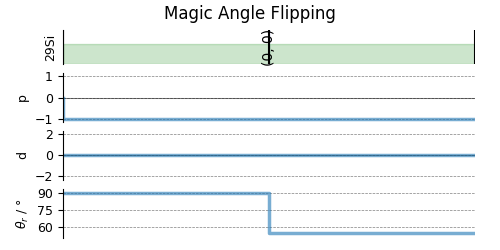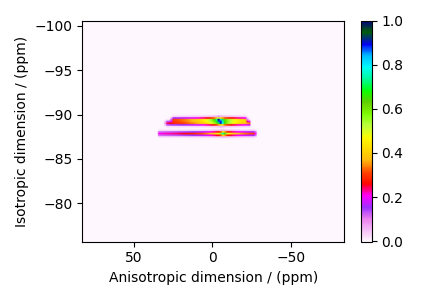# Wollastonite, ²⁹Si (I=1/2), MAF¶

²⁹Si (I=1/2) magic angle flipping.

Wollastonite is a high-temperature calcium-silicate, $$\beta−\text{Ca}_3\text{Si}_3\text{O}_9$$, with three distinct $$^{29}\text{Si}$$ sites. The $$^{29}\text{Si}$$ tensor parameters were obtained from Hansen et al. 1

import numpy as np
import matplotlib.pyplot as plt

from mrsimulator import Simulator, SpinSystem, Site
from mrsimulator import signal_processor as sp
from mrsimulator.spin_system.tensors import SymmetricTensor
from mrsimulator.method import Method, SpectralDimension, SpectralEvent, MixingEvent


Create the sites and spin systems

sites = [
Site(
isotope="29Si",
isotropic_chemical_shift=-89.0,  # in ppm
shielding_symmetric=SymmetricTensor(zeta=59.8, eta=0.62),  # zeta in ppm
),
Site(
isotope="29Si",
isotropic_chemical_shift=-89.5,  # in ppm
shielding_symmetric=SymmetricTensor(zeta=52.1, eta=0.68),  # zeta in ppm
),
Site(
isotope="29Si",
isotropic_chemical_shift=-87.8,  # in ppm
shielding_symmetric=SymmetricTensor(zeta=69.4, eta=0.60),  # zeta in ppm
),
]

spin_systems = [SpinSystem(sites=[s]) for s in sites]


Use the generic Method class to simulate a 2D magic-angle Flipping (MAF) spectrum by customizing the method parameters, as shown below.

Here, we include a MixingEvent with a NoMixing query. A no mixing query instructs the MAF method to not mix the transitions from the first and second SpectralEvent. A no mixing query is equivalent to a rotation query where each channel has a zero phase and angle. Since all spin systems in this example have a single site, defining no mixing between the two spectral events is superfluous. We include it such that the method is applicable with multi-site spin systems.

maf = Method(
name="Magic Angle Flipping",
channels=["29Si"],
magnetic_flux_density=14.1,  # in T
rotor_frequency=np.inf,
spectral_dimensions=[
SpectralDimension(
count=128,
spectral_width=2e4,  # in Hz
label="Anisotropic dimension",
events=[
SpectralEvent(
rotor_angle=90 * np.pi / 180,  # in rads
transition_queries=[{"ch1": {"P": [-1], "D": }}],
),
MixingEvent(query="NoMixing"),
],
),
SpectralDimension(
count=128,
spectral_width=3e3,  # in Hz
reference_offset=-1.05e4,  # in Hz
label="Isotropic dimension",
events=[
SpectralEvent(
rotor_angle=54.735 * np.pi / 180,  # in rads
transition_queries=[{"ch1": {"P": [-1], "D": }}],
)
],
),
],
affine_matrix=[[1, -1], [0, 1]],
)

# A graphical representation of the method object.
plt.figure(figsize=(5, 2.5))
maf.plot()
plt.show()Create the Simulator object, add the method and spin system objects, and run the simulation.

sim = Simulator(spin_systems=spin_systems, methods=[maf])
sim.run()


csdm_dataset = sim.methods.simulation
processor = sp.SignalProcessor(
operations=[
sp.IFFT(dim_index=(0, 1)),
sp.apodization.Gaussian(FWHM="50 Hz", dim_index=0),
sp.apodization.Gaussian(FWHM="50 Hz", dim_index=1),
sp.FFT(dim_index=(0, 1)),
]
)
processed_dataset = processor.apply_operations(dataset=csdm_dataset).real
processed_dataset /= processed_dataset.max()


The plot of the simulation after signal processing.

plt.figure(figsize=(4.25, 3.0))
ax = plt.subplot(projection="csdm")
cb = ax.imshow(processed_dataset.T, aspect="auto", cmap="gist_ncar_r")
plt.colorbar(cb)
ax.invert_xaxis()
ax.invert_yaxis()
plt.tight_layout()
plt.show()1

Hansen, M. R., Jakobsen, H. J., Skibsted, J., $$^{29}\text{Si}$$ Chemical Shift Anisotropies in Calcium Silicates from High-Field $$^{29}\text{Si}$$ MAS NMR Spectroscopy, Inorg. Chem. 2003, 42, 7, 2368-2377. DOI: 10.1021/ic020647f

Total running time of the script: (0 minutes 0.660 seconds)

Gallery generated by Sphinx-Gallery